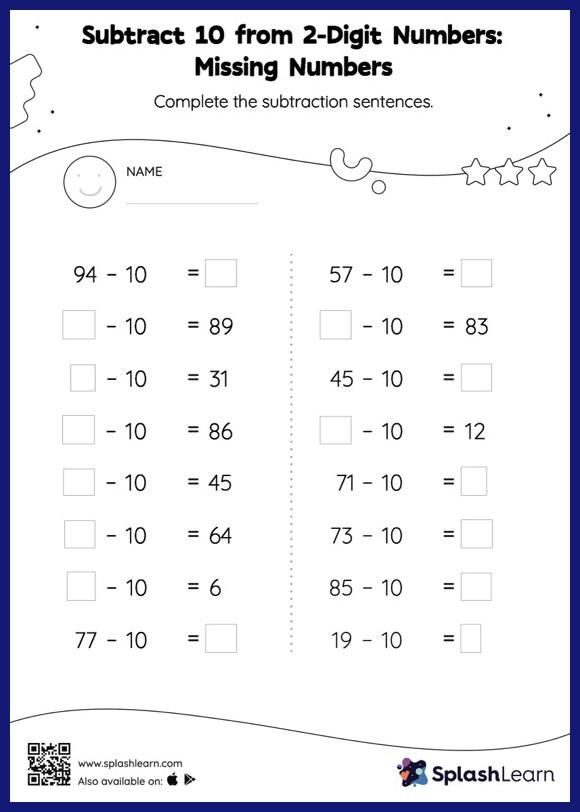# Subtract 10 from 2-Digit Numbers: Missing Numbers Worksheet

Home > Subtract 10 from 2-Digit Numbers: Missing NumbersThis worksheet consists of a set of questions on subtraction where students are required to subtract 10 from 2-digit numbers. Students use the count-back method or the relationship between addition and subtraction to find the missing number if it is a minuend or subtrahend. Also, they subtract the numbers to get the difference if the missing number is the difference. There is a lot of practice with this concept in the worksheet subtract 10 from 2-digit numbers.